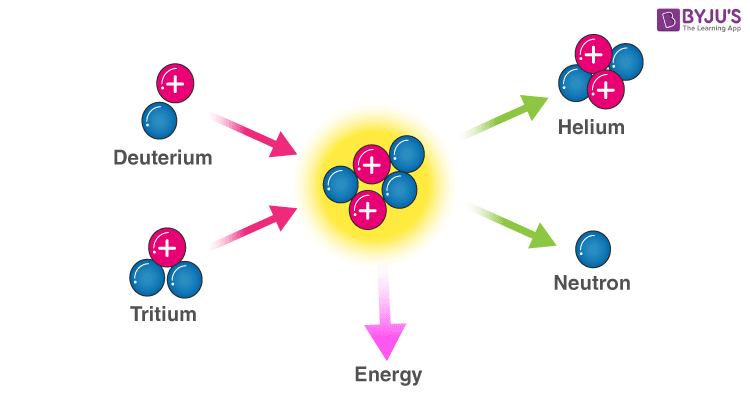Checkout JEE MAINS 2022 Question Paper Analysis : Checkout JEE MAINS 2022 Question Paper Analysis :

# Power And EnergyWe often consider Power and Energy to be the exact synonyms. Energy and power are said to be closely related but not the same type of physical quantities. Though they are used in everyday life, the difference between them is fine but significant.

Energy is the capacity to do some physical activities or work, such as running, jumping, etc., while power is defined as the rate at which the energy is transferred, or the work is completed. The unit used to measure energy is joules, ergs and calories. Power is measured in watts. Wherein in other terms, it is ergs per second or even joules per second.

Let us consider the example of a weightlifter to understand the concept of Energy and Power more precisely. Power is something like the strength of a weightlifter, whereas energy is said to measure how long he could sustain that power output. Energy is ‘joules’, and power is the ‘joules per second’.

Energy changes from one form to another, but not the power. If something has to happen, Energy is said to change its form. Power is known only to measure how fast the change has occurred; in other words, power is defined as the rate at which energy converts every second.

## Difference between Energy and Power

 Sr.no Energy Power 1. Energy is defined as the capacity to do some work. It is the power which is integrated over time. Power is defined as the rate at which specific work is done or which the energy is transmitted. 2. The unit used to measure energy is joules or watt-seconds The unit used to measure this is watt or joules per second 3. ‘W’ is the symbol which denotes energy The symbol used to denote power is ‘P’. 4. Energy changes from one form to another Power cannot be transformed from one type to another 5. Energy is a time quantity or component It is an instantaneous quantity 6. Various types of energy are kinetic, thermal, potential, gravitational, sound, electromagnetic, light, elastic, etc Different kinds of power are electric power, optical power, human power, etc. 7. Energy is known to be stored, which can be used in the future Power quantity is not storable or cannot be stored 8. Energy is used in moving a car, heating a home, lighting at night, flying an aeroplane, etc. Power finds its uses in mechanical applications, electrical applications, heat applications, etc.

## Frequently Asked Questions – FAQs

### What is power?

Power is defined as the rate at which specific work is done, or which the energy is transmitted.

### What is energy?

Energy is defined as the capacity to do some work. It is the power which is integrated over time.

### What are the two main types of energy?

Potential energy and kinetic energy are the two main types of energy.

### What are the uses of energy?

Energy is used in moving a car, heating a home, lighting at night, flying an aeroplane, etc.

### What is the unit of power?

The unit used to measure power is watt or joules per second.

Stay tuned to BYJU’S and Keep Falling in Love with Learning!

Test your knowledge on Power And Energy Difference